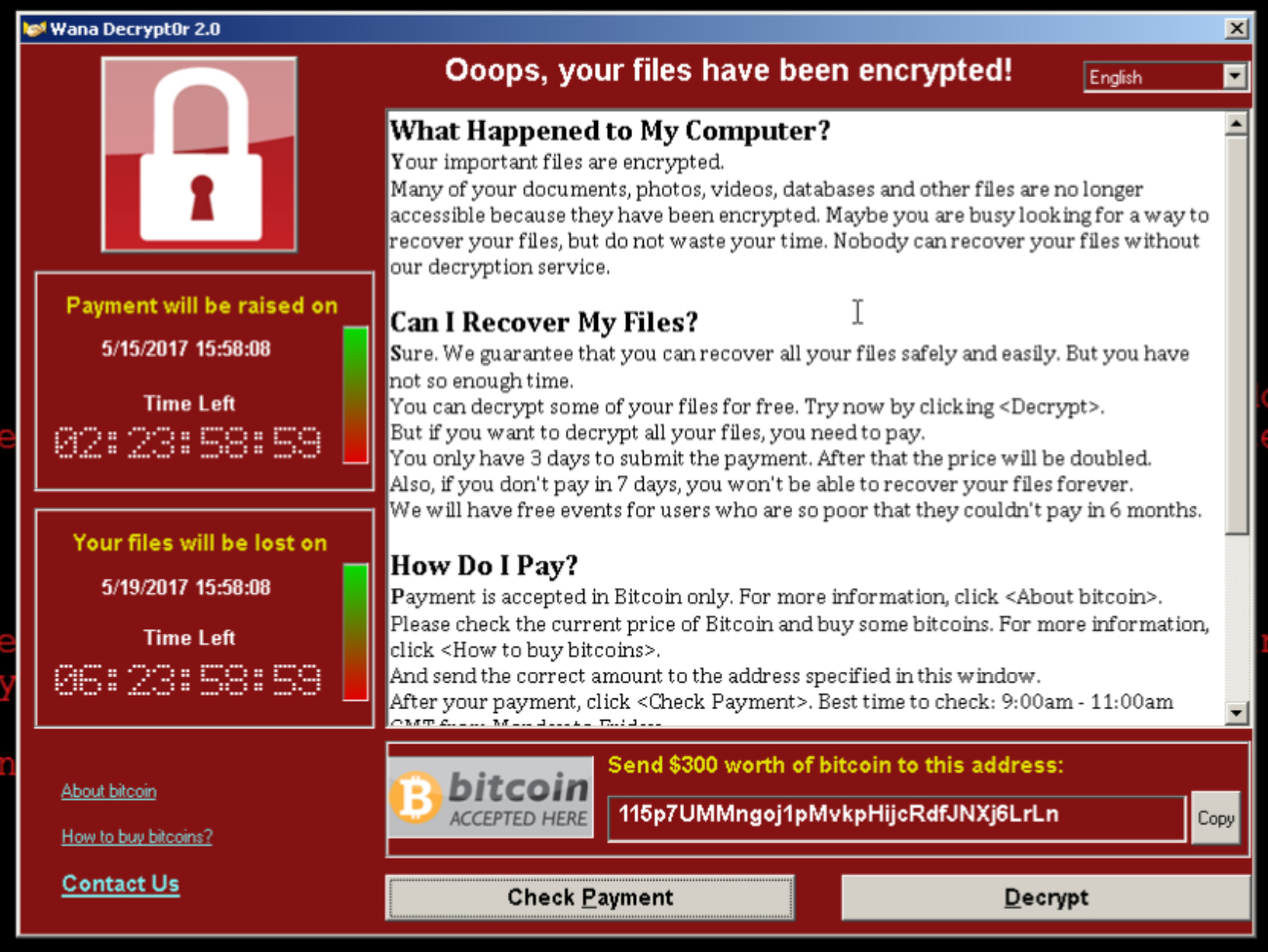# RSA加密

2017年五月13日早些时候群里就流传了一些学校机房里的机器中了一种蠕虫病毒的图，那时候都是怀着一种调侃的心情看这些图，因为机房没法使用我们学生们就不用上一些实习了233。WannaCry会加密硬盘上的所有文件，它还会提示你，如果你想解密，需要给一个比特币地址支付比特币。那么它怎么确认是谁交了比特币呢？它得确保每个被感染的机器都有唯一的标识，不能用一个统一的比特币钱包地址，因为这样没法确认是谁交了比特币。WannaCry确实会通过tor网络给每一个感染机器分配一个比特币地址，这样作者只需要给检测单独比特币钱包是否有足够的比特币就可以给那些机器解密密钥。但是在中国的GFW是屏蔽了tor网络的。所以在中国地区感染的机器只会显示三个固定的比特币地址

115p7UMMngoj1pMvkpHijcRdfJNXj6LrLn

12t9YDPgwueZ9NyMgw519p7AA8isjr6SMw

13AM4VW2dhxYgXeQepoHkHSQuy6NgaEb94

 1 2 3   BigInteger q = BigInteger.probablePrime(100, new Random()); // 取 2^100-1 范围内的随机素数 BigInteger p = BigInteger.probablePrime(100, new Random()); BigInteger N = q.multiply(p); // q * p 

 1 2  BigInteger r = q.subtract(BigInteger.ONE).multiply(p.subtract(BigInteger.ONE)); //(q - 1)*(p - 1) 

openssl的低级版本一般会将e=3作为默认的RSA公钥指数。选取小公钥指数主要是为了提高加密或签名验证的性能，比如选e=3只需要2次模乘(ModMul)，而随机选择的e(假设n是1024-bit)则大概需要1000次模乘。NIST SP800-78 Rev 1 (2007) 曾强调“不允许使用比65537更低的公钥指数e”，但PKCS#1却从未有过类似的建议。e=65537=(2^16+1)，其签名/加密运算只需要17次模乘，性能也算不错。但我认为选这个值的目的只是一个介于小指数攻击和运算效率之间的一个折中考虑，即以防万一将来有一天"e=3"被攻破而侥幸"e=65537"可能还是安全的。

（http://blog.csdn.net/hherima/article/details/52461759）

java里定义了静态变量用于exgcd使用，这里写得很不好看。。。或许还有更优雅的写法。

  1 2 3 4 5 6 7 8 9 10 11 12 13  static BigInteger x,y,o = BigInteger.valueOf(0),o1 = BigInteger.valueOf(1); public static BigInteger exgcd(BigInteger a,BigInteger b){ if(b.compareTo(o)==0){ x = o1; y = o; return a; }else{ BigInteger d = exgcd(b,a.mod(b)); BigInteger temp = x; x = y; y = temp.subtract(a.divide(b).multiply(y)) ; return d; } } 

 1  BigInteger n = new BigInteger("65535"); 

 1 2 3   BigInteger n = new BigInteger("65535"); System.out.println("n= " + n); BigInteger c = n.modPow(e, N); 

 1 2 3   System.out.println("c= " + c); BigInteger nc = c.modPow(d, N); System.out.println("nc= " + nc);，即。 由欧拉定理得：RSA同样可以用来签名消息，只要拥有私钥的一方在消息后添加消息的散列值的加密信息，拥有公钥的一方接收到消息后可以用公钥解密散列值并于消息对比，如果消息的散列值与解密后的一致，则这个消息在传播路径上没有被篡改过。

  1 2 3 4 5 6 7 8 9 10 11 12 13 14 15 16 17 18 19 20 21 22 23 24 25 26 27 28 29 30 31 32 33 34 35 36 37 38 39 40 41 42 43 44 45 46 47 48  package math; import java.math.*; import java.util.Random; public class Encode { static BigInteger x,y,o = BigInteger.valueOf(0),o1 = BigInteger.valueOf(1); public static void main(String[] args) { // TODO 自动生成的方法存根 BigInteger q = BigInteger.probablePrime(100, new Random()); // 取 2^100-1 范围内的随机素数 BigInteger p = BigInteger.probablePrime(100, new Random()); BigInteger N = q.multiply(p); // q * p BigInteger r = q.subtract(BigInteger.ONE).multiply(p.subtract(BigInteger.ONE)); //(q - 1)*(p - 1) System.out.println("q= " + q); System.out.println("p= " + p); System.out.println("N= " + N); System.out.println("r= " + r); BigInteger e = new BigInteger("65537" );//new BigInteger(10,new Random()); //while(r.gcd(e).equals(BigInteger.ONE)){ //System.out.println(e); //e =new BigInteger(10,new Random()); // } System.out.println("e= " + e); exgcd(e, r); BigInteger d = x;//new BigInteger("2753" ); System.out.println("d= " + d); //BigInteger n = BigInteger.probablePrime(11, new Random()); BigInteger n = new BigInteger("65535"); System.out.println("n= " + n); BigInteger c = n.modPow(e, N); System.out.println("c= " + c); BigInteger nc = c.modPow(d, N); System.out.println("nc= " + nc); } public static BigInteger exgcd(BigInteger a,BigInteger b){ if(b.compareTo(o)==0){ x = o1; y = o; return a; }else{ BigInteger d = exgcd(b,a.mod(b)); BigInteger temp = x; x = y; y = temp.subtract(a.divide(b).multiply(y)) ; return d; } } }